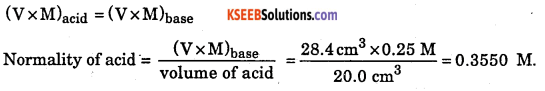# 1st PUC Chemistry Question Bank Chapter 1 Some Basic Concepts of Chemistry

## Karnataka 1st PUC Chemistry Question Bank Chapter 1 Some Basic Concepts of Chemistry

### 1st PUC Chemistry Some Basic Concepts of Chemistry One Mark Questions and Answers

Question 1.
What is the unit for equivalent weight?
Equivalent weight is a mere number, it has no units. When expressed in grams it is called gram equivalent weight.

Question 2.
Define Atomic weight.
The atomic weight of an element is the ratio of average mass of an atom of an element to $$\frac{1}{12}$$ of mass of an atom of C-12 Isotope.

Question 3.
Write the mathematical form of Atomic weights.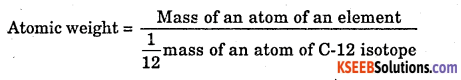Question 4.
Mention the unit for equivalent weight.
There is no unit for atomic weight, when expressed in grams it is called gram atomic weight.Question 5.
Write the Relationship between Atomic weight and Valency weight.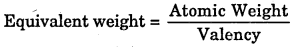Question 6.
Define Valency.
Valency: The combining capacity of an atom (or the number of electrons present in the outermost orbital of an atom which involves in bond formation.

Question 7.
Mention the methods to determine Equivalent weight.

• Oxide Method
• Chloride Method
• Hydrogen Displacement method
• Interconversion method.

Question 8.
Define Molecular weight.
It is defined as “ The ratio of the mass of 1 molecule of the substance to $$\frac{1}{12}$$ mass of an atom of C-12 isotope”.Question 9.
Write mathematical forms of Molecular weight.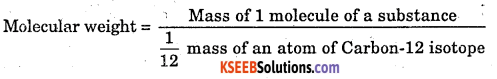Question 10.
Equal volume of all gases and vapours under the same condition of temperature and pressure contains an equal number of molecules.

Question 11.
Define Mole.
1 mole represents the amount of substance which contains an Avagadro number of particles.

Question 12.
What do you mean by Avagadro Number?
The number of molecules present in 1 gm molecular weight of any substance.
OR
The number of atoms present in 1 gm atomic weight of any element. It is represented by the symbol N = 6.022 × 1023

Question 13.
Define Molality.
The number of moles of solute dissolved in 1 kg of the solution.Question 14.
Define Molarity.
A number of the molecular equivalent of solute dissolved in 1 dm3 of the solution.

Question 15.
What is mole fraction?
The ratio of the number of moles of a component to the number of moles present in the solution.

Question 16.
What do you mean by a Normal solution?
A solution containing 1 gm equivalent of the substance dissolved in 1 dm3 of the solution.

Question 17.
What do you mean by the decinormal solution?
A decinormal solution is the solution containing 0.1 g equivalent ($$\frac { 1 }{ 10 }$$ th) of solute / substance dissolved in 1 dm3 of solution.

Question 18.
What are indicators?
Indicators are the chemical substance used to indicate the endpoint of a volumetric reaction by the change in colour.

Question 19.
Mention the types of Indicators.

1. Internal indicator
2. External indicator
3. Self indicatorFormula of normality The Normality is defined as the number of gram equivalent of the solute dissolved per litre of the solution.

Question 20.
Define empirical formula.
It is a formula which gives the relative number of atoms of each element in the compound.

Question 21.
Define Molecular formula.
It is a formula which gives the actual number of atoms of each element present in the molecule of the given compound.

Question 22.
What is the relation between empirical and molecular formula?Question 23.
State the law of definite proportion.
The law states that “a chemical compound, however, prepared, contains the same elements combined together in the same fixed proportion by mass”.

Question 24.
Define the law of conservation of mass.
It states that matter can neither be, created nor be destroyed.

Question 25.
State Gay Lussac’s law of combining volumes.
The law states that ’when gaseous reactants combine to give gaseous products, they do so in volumes which bear a simple whole number ratio to one another and to the volume of products, provided the volumes are measured under similar conditions of temperature and pressure”.

Question 26.
What is NTP?
(Normal temperature and pressure or standard temperature and pressure). The temp 273K (0 °C) and pressure 101.3Kpa (or N/m2) or 1 atm = 760 mm of Hg level are taken as STP or NTP.Question 27.
What physical quantities are represented by the following units and what are their most common names?
(i) kg ms-2
(ii) kg m2s-2
(iii) dm3
(i) Force (newton)
(ii) Work (joule)
(iii) Volume

Question 28.
Rewrite the following after required corrections:
(i) The length of a rod is 10 cms
(ii) the work done by a system is 10 Joules.
(i) The length of a rod is 10 cm (s is not used)
(ii) The work done by a system is 10 joules (the small letter is used in place of the capital letter).Question 29.
Classify the following substances into elements, compounds, and mixtures:
(i) Milk
(ii) 22-carat gold
(iii) Iodized table salt
(iv) Diamond
(v) Smoke
(vi) Steel
(vii) Brass
(viii) Dry ice
(ix) Mercury
(x) Air
(xi) Aerated drinks
(xii) Glucose
(xiii) Petrol/Diesel/Kerosene oil
(xiv) Steam
(xv) Cloud.
Element – (iv), (ix);
Compounds – (viii), (xii), (xiv), (xv);
Mixtures – (i), (ii), (iii), (v), (vi), (vii), (x), (xi), (xiii).

Question 30.
Why is air sometimes considered a heterogeneous mixture?
This is due to the presence of dust particles which change the phase, into heterogeneous.Question 31.
1 L of a gas at STP weighs 1.97 g. What is the vapour density of the gas?
22.4 L of the gas at STP will weigh 1.97 × 22.4 = 44.1 g ie., molecular mass = 44.1.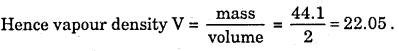Question 32.
Why atomic masses are the average values?
Most of the elements exist in different isotopes ie., atoms with different masses, eg. Cl has two isotopes with mass numbers 35 and 37 existing in the ratio 3:1. Hence, the average value is taken.

Question 33.
Determine the equivalent weight of each of the following compounds assuming the formula weights of these compounds are x, y, and z respectively, (i) Na2SO4 (ii) Na3P04.12H2O (iii) Ca3(PO4)2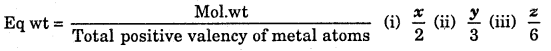Question 34.
What is the mass of mercury in grams and in kilograms if the density of liquid mercury is 13.6 g cm-3?
Mass = Volume x density = 1000 cm3 × 13.6 g cm-3 = 13,600 g = 13.6 kg

Question 35.
Vitamin C is known to contain 1.29 × 1024 hydrogen atoms. Calculate a number of moles of hydrogen atoms.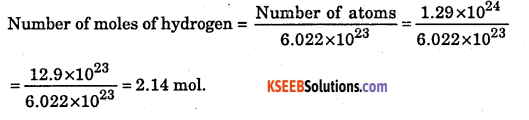Question 36.
Define an organic compound. Give one example.
An organic compound contains essentially carbon, hydrogen along with certain elements like N, P, S, O, halogen, etc., eg: urea, sugar.

Question 37.
Define an inorganic compound. Give one example.
An inorganic compound is made up of two or more elements containing a rare percentage of carbon eg: NaCl, KC1, ZnS, etc.

Question 38.
Name the process used for refining petroleum.
Fractional distillation.

Question 39.
Calculate the percentage of nitrogen in NH4. (Atomic mass of N = 14, H = 1 amu)Question 40.
State the law of multiple proportion.
It states ‘whenever two elements combine to form two or more compounds, the ratio between different weights of one of the elements which combines with fixed weight of other is always simple’.Question 41.
Calculate the number of He atoms in (i) 52 u, (ii) 52 g, (iii) 52 moles of He. Atomic weight of He is 4 u.
(i) Atomic weight of Helium = 4, i.e. 4 u is mass of 1 atom,
52 u is mass of $$\frac { 1 }{ 4 }$$ × 52 = 13 atoms.

(ii) 4 g of He contains 6.022 × 1023 He atoms / 52 g of He contains ?
$$\frac{6.022 \times 10^{23} \times 52}{4}$$ = 7.8286 × 1023 atoms.

(iii) 1 mole of He contains 6.022 × 1023 atoms, 52 moles of He contains 52 × 6.022 × 1023 =3.131 × 1023 atoms.

Question 42.
How many electrons are present in 16 g of CH4 ?
1 molecule of CH4 =6 + 4 = 10 electrons, 16 g of CH4 contains 10 × 6.022 × 1023 = 6.022 × 1024

Question 43.
Boron occurs in nature in the form of two isotopes and $$\begin{array}{l}{11} \\ {5}\end{array}$$ B and $$\begin{array}{l}{10} \\ {5}\end{array}$$ in ratio of 81% and 19% respectively. Calculate its average atomic mass.Question 44.
If 2 litres of N2 is mixed with 2 litres of H2 at a constant temperature and pressure. What will be the volume of NH3 formed?
N2(g) + 3H2(g) → 2NH3(g)Question 45.
How many atoms are present in 4 ml of NH3 at STP?
22400 ml of NH3 contains = 4 × 6.022 × 1023 atoms [∵ NH3 contains 4 atoms]. 1ml of NH3 contains = $$\frac{4 \times 6.022 \times 10^{23}}{22400}$$ = 1.07 × 1020 atoms.

Question 46.
Which of these weighs most? (i) 32 g of oxygen, (ii) 2 g atom of hydrogen, (iii) 0.5 mole of Fe, (iv) 3.01 × 1023 atoms of carbon.
(i) 32 g of oxygen weighs most.
(ii) 2 g atom of H2 = 2g
(iii) 0.5 mole of Fe = mole × atomic weight = 0.5 × 56 = 28 g
(iv) 6.022 × 1023 = 1 atom of d 3.01 × 1023 atoms of C = $$\frac { 1 }{ 2 }$$ × 12 = 6 g.

Question 47.
An element has a specific heat of 0.113 cal/g °C. Calculate atomic weight of element.
According to Dulong Petitt’s law, Atomic weight × Specific heat = 6.4 cal
Atomic weight × 0.113 cal/g = 6.4 cal
Atomic weight = $$\frac { 6.4 }{ 0.113 }$$ = 56Question 48.
Why are the atomic masses of most of the elements fractional?
This is because atomic masses are the relative masses of atoms as compared with an atom of C-12 isotope taken as 12.

Question 49.
Match the following:A-(ii)
B-(iii)
C-(iv)
D-(i)

Question 50.
Match the following prefixes with their multiplies.(i) micro 10-6
(ii) deca – 10
(ill) mega – 106
(iv) giga – 109
(v) femto – 10-15

Question 51.
Calculate the molar mass of the following:
(i) H2O (ii) CO2 (iii) CH4
(i) H2O = 2 × H+ 1 × O
2 × 1 + 1 × 16 = 18
(ii) CO2 = 12 × 1 + 2 × 16 = 44
(iii) CH4 = 1 × C + 4 × H
1 × 12 + 4 × 1 =16

### 1st PUC Chemistry Some Basic Concepts of Chemistry Two Marks Questions and Answers

Question 1.
Define the basicity of an acid with an example.
Basicity of an acid is the number of replaceable hydrogen atoms present on a molecule of acid is called the basicity of an acid.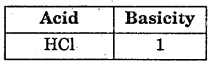Question 2.
Define acidity of a base with example.
The acidity of a base is the number of monobasic acids required to neutralize 1 molecule of a base. [The base which contains a number of replaceable – OH group].Question 3.
Define equivalent weight of acid with example.
The number of parts by mass of the acid contains 1 part by mass of replaceable hydrogen.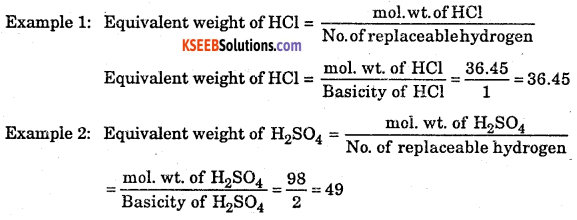Question 4.
Calculate the equivalent weight of the following acid, (a) H2C2O4.H2O (b) H2O2O4Question 5.
Define equivalent weight of base with an example.
NaOH + HCl → NaCl + H2O
1 equivalent of HC1 = 36.45, here 1 equivalent of HCl reacts with NaOH.Question 6.
Write the acidity and calculate the equivalent weight of the following base, a) Ca(OH)2 b) Fe(OH)3Question 7.
Write the mathematical form for mole fraction.
Mathematically;
If n1 is the number of moles of solvent; If n2 is the number of moles of solute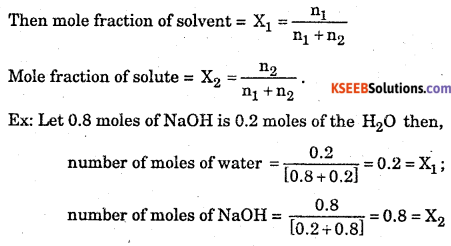Question 8.
What is ppm (parts per million)?
It is the mass of solute present in 1 million(104) of solution.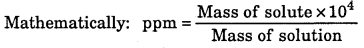10-3 = 1 mg of solute /1 dm3 of solution.
∴ 1 ppm = 1 mg of solute /1 dm3 of solution.
n ppm = n mg solute / 1 dm3 of solution.

Question 9.
Define Normality.
Number of gram equivalent of the substance dissolved in 1 dm3 of solution.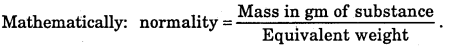Question 10.
Calculate molarity and normality of the following solution, a) HCl b) H2C2O4Question 11.
Write a note on the internal indicator.
The internal indicator is the substance which is added to the titration flask at the beginning. Example: Methyl orange, starch, phenolphthalein, diphenylamine.
Internal indicators are further divided according to the type of reaction taking place during titration.

(a) Acid-base indicator: The choice of indicator for acid-base titration depends on the nature of the acid and base used in that reaction.
Acid-Base Indicator
Strong acid Strong base Methyl orange or phenolphthalein(b) Redox indicator: Example: Diphenylamine. When titrating KMnO4 with oxalic acid.

Question 12.
What are external indicators? Give an example.
The external indicator is added in between the titration process by taking a drop of titrated
mixture.
Example: Potassium ferrocyanide is used when ferrous ammonium sulphate is titrated against K2Cr2O7.

Question 13.
What is the self indicator? Give an Example.
When in titration one of the solutions acts as a self indicator. Example: KMnO4.Question 14.
What is gram molecular value? Explain.
The volume occupied by one gram mole of a substance in the gaseous or is the vapour state at STP is called the gram molecular volume. The gram molecular volume of all the substances is found to be 22.4 dm 3 or 22400 cm 3. The mass in gram of 22.4 dm 3 of a gas or vapour at STP is equal to its gram molecular weight.

1 g molecule of chlorine or 1 gram molecule of carbon-di-oxide or 1 gram of any other substance in the gaseous state occupies a volume of 22400 cm3 (22.4 dm3 ).

1 g molecule weight of hydrogen = 2.016 g, 2.016 g of hydrogen occupies 22.4 dm3 of volume at STP. But 1 g equivalent weight of hydrogen is 1.008 g occupies 11.2 dm3 of volume at STP or mass of 11200 cm 3 of hydrogen = its equivalent weight.

Question 15.
Define the following with examples, (i) Allotropy and allotropes, (ii) polymorphism, (iii) isomorphism.
(i) The existence of an element in two or more chemically similar but physically different forms is called allotropy and the different forms are called allotropes, e.g., diamond, graphite, wood charcoal, lamp black etc., are allotropes of carbon.
(ii) The existence of a compound in different crystalline forms is called polymorphism and the different forms are called polymorphs, e.g., ZnS has two polymorphs called zinc blende and wurtzite.
(iii) The existence of different compounds with similar chemical composition in the same crystalline form is called [M2SO4M2 (SO4)3 24H2O] are isomorphs.

Question 16.
Discuss smaller the quantity to be measured more precisely should be the instrument.
Quite often the uncertainty in measurement is expressed in terms of percentage by putting ± sign before it, e.g., 250 ± 1%, etc. If the same instrument is used for measuring different quantities, then smaller the quantity to equal to ± 1 mg, then if we weigh 100 g on it, the result can be reported as 100 ± 0.001%. If the same balance is used to weigh 10 g, the result reported will be 10 ± 0.01%, and if 1 g is weighed, the result reported will be 10 ± 0.1%. Hence, the smaller the quantity to be measured, the more precise should be the instrument.

Question 17.
Compute the mass of one molecule and the molecular mass of C6H6  (benzene). (At. mass of C = 12, H = 1 u).
Molecular weight of C6H6 = 6 × 12 + 6 × 1 = 78 g,
Mass of 1 molecule = $$\frac{78}{6.002 \times 10^{23}}$$ = 12.94 × 10-23 g = 1.294 × 10-22 g

Question 18.
An organometallic compound on analysis was found to contain, C = 64.4%, H = 5.5% and Fe = 29.9%. Determine its empirical formula. (At. mass of Fe = 56 u).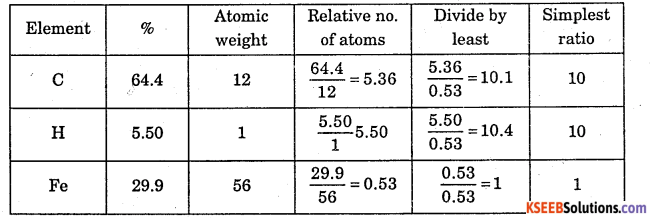Question 19.
4g of copper chloride on analysis was founded to contain 1.890 g of copper (Cu) and 2.110 g of chlorine (Cl). What is the empirical formula of copper chloride? [At. mass of Cu = 63.5 u, Cl = 35.5 u].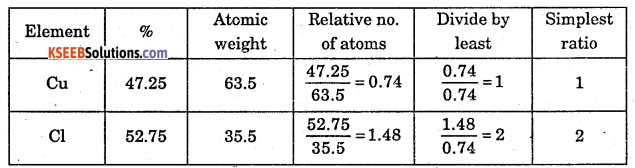Question 20.
Calculate the number of grams of oxygen in 0.10 mol of Na2CO3.10H2O.
1 mole of Na2CO3.10H2O contains 13 moles of oxygen atoms.
0.1 mole Na2CO3.10H2O contains 13 × 0.1 = 1.3 moles of oxygen atoms.
Mass of oxygen atoms = 1.3 × 16 = 30.8 g.

Question 21.
How many grams of Cl2 are required to completely react with 0.4 g of H2 to yield HC1? Also, calculate the number of HCl formed.
H2(g) + Cl2(g) → HCl(g) ,
2 × 1 = 2
2 × 35.5 = 71
2 g of H2 reacts with 71 g of Cl2 to give 73 g of HCl.
0.4 g of H2 reacts with $$\frac{71}{2}$$x 0.4 = 14.2 g of Cl2 to give $$\frac{73 \times 0.4}{2}$$ = 14.6 g of HCl.
14.2 g of Cl2 and 14.6 g of HCl.

Question 22.
Cone. HC1 is 38% HCl by mass. What is the molarity of this solution if d = 1.19g cm-3? What volume of conc. HCl is required to make 1.00 L of 0.10 M HCl?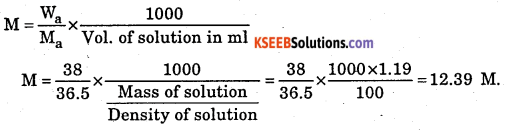Question 23.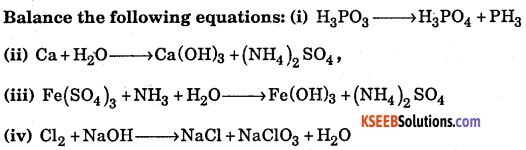Question 24.
Calculate the volume of O2 at STP liberated by heating 12.25 g of KClO3. (At. wt. of K = 39, Cl = 35.5, O = 16 u).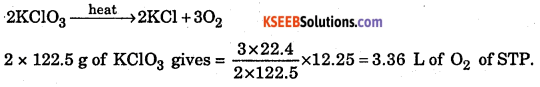Question 25.
1 M solution of NaNO3 has a density of 1.25 g cm -3. Calculate its molality. (Mol. weight of NaNO3 = 85 g mol-1).
Mass of solution = Volume of solution × density of the solution
Mass of solution = 1000 cm3 × 1.25 g cm-3 = 1250 g
Mass of solute = 85 g; Mass of solvent = 1250 – 85 = 1165 g.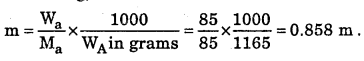Question 26.
Explain how compounds differ from elements? Give two differences.
Elements consist of only one kind of atom.Question 27.
Explain how mixture differs from pure substance? Give 2 differences.
Pure substances Mixtures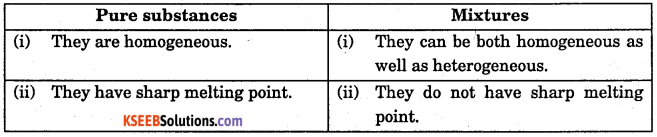Question 28.
Classify each of the following as pure substance or mixture:

1. Ethyl alcohol
2. Oxygen
3. Blood
4. Carbon
5. Steel
6. Distilled water.

Pure substances:

1. Ethyl alcohol
2. Oxygen
3. Carbon
4. Distilled water

Mixtures:

1. Blood
2. Steel

Question 29.
Describe two factors that introduce uncertainty into measured figures.

1. Reliability of measuring instrument
2. Skill of the person making the measurement.

Question 30.
A 50 ml graduated cylinder has 1 ml graduation. What is the maximum number of significant figures of the volumes that can be reported from this graduated cylinder?
The maximum number of significant figures of the volumes that can be reported is 2.

Question 31.
What is the difference between the accuracy and precision of measurements?
Accuracy: It is related to the closeness of a signal measurement to its true value.
Precision: It refers to the closeness of the set of values obtained from identical measurements of a quantity.

Question 32.
Classify the following substances into elements, compounds, and mixture.
(1) Milk (2) Iodised table salt (3) Diamond (4) Steel (5) Dry Ice (6) Glucose (7) Petrol (8) Steam (9) Cloud (10) Aerated drinks
Elements (3), Compounds 5,6, 8,9, Mixture 1, 2,4, 7,10### 1st PUC Chemistry Some Basic Concepts of Chemistry Five Marks Questions and Answers

Question 1.
Calculate the moles of NaOH required to neutralize the solution produced by dissolving 1.1 g P4O6 in water. Use the following reactions:
P4O6 + 6H2O → 4H3PO3;
2NaOH + H3PO3 → Na2HPO3 + 2H2O
(At. mass/g mol-1 P = 31, O = 16).
Molecular weight of P4O6 =4 × 31 + 6 × 16 =124 + 96 = 220 gmol-1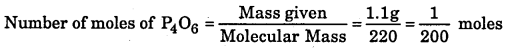$$\frac { 1 }{ 200 }$$ moles of PiOfi produce 4 × $$\frac { 1 }{ 200 }$$moles of H3PO3
1 mole of H3PO3 requires 2 moles of NaOH
$$\frac { 1 }{ 50 }$$moles of H3PO3 requires 2 × $$\frac { 1 }{ 50 }$$ = $$\frac { 1 }{ 25 }$$ = 0.04 moles of NaOH.

Question 2.
(a) A sample of NaOH weighing 0.38 g is dissolved in water and the solution is made to 50.0 cm3 in a volumetric flask. What is the molarity of the solution?
(b) State and explain law of multiple proportion.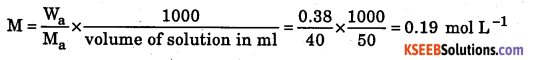(b) Law of multiple proportion states whenever two elements react to form two or more compounds, the ratio between different weights of one of the elements which combine with fixed weight of another is always simple, e.g., nitrogen reacts with oxygen to form NO and NO2. In NO, 14 g of N reacts with 16 g of O. In NO2,14 g of N reacts with 32 g of O. The ratio between weights of oxygen which combine with fixed weight of nitrogen is 16 : 32. i.e., 1:2.

Question 3.
Calculate the amount of water (g) produced by the combustion of 16 g of methane.
CH4(g) + 2O2(g) >CO2(g) + 2H2O(1)
1 mole = 16 g of CH4 gives 2 moles of H2O i.e., 2 × 18 = 36 g of H2O.

Question 4.
How many moles of methane are required to produce 22 g of CO,2(g) after combustion?
44 g of CO2 will be produced by 1 mole of CH4.
22 g of CO2 will be formed by $$\frac{1}{44}$$ × 22 = 0.5 mole of CH4.Question 5.
(a) How many significant figures are there in 1.00 × 106?
(b) One mole of sugar contains ……. oxygen atoms.
(c) Give an example of a molecule in which the empirical formula is CH2O and the ratio of molecular formula weight and empirical formula weight is 6.
(a) 3
(b) One mole of sugar C12H22O11 contains 11 × 6.023 × 1023 oxygen atoms.
= 66.253 × 10 23 = 6.6253 × 10 23 atoms of oxygen
(c) C6H12O6.

Question 6.
An organic monobasic acid was found to contain 39.5% carbon, 6.4% hydrogen and the rest oxygen. If the equivalent mass of the acid is 60, find out its molecular formula.
Percentage of oxygen = 100 – (39.5 + 6.4) = 100 – 45.9 – 54.1∴Empirical formula = CH2O
Molecular mass = Eq. mass × basicity = 60 × 1 = 60
Empirical formula weight = CH20 = 12 + 2 + 16 = 30
Nows 30n = 60 ⇒ n = 2
∴Molecular formula = (Empirical formula) × n = (CH2O)2 = C2H4O2

Question 7.
Calculate the mass of 95% pure MnO2 to produce 35.5 g of Cl2 as per the following reaction.
MnO2 + 4HCl → MnCl2 + Cl2 + H2O.
(At. mass of Mn = 55)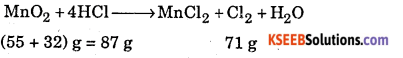71 g of Cl2 is produced by 87 g of MnO2
35.5 g of Cl2 is produced by $$\frac { 87 }{ 71 }$$ × 35.5 = 43.49 g of MnO2
Since MnO2 is 95% pure i.e., 95 g of MnO2 is present in 100 g of MnO2 sample.
43.49 of pure MnO2 will be present in $$\frac{43.49 \times 100}{95}$$= 45.8f of MnO2 sample

Question 8.
500 ml of Na2CO3 solution contains 2.65 g of Na2CO3 (mol. mass of Na2CO3 = 106). If 10 ml of this solution is diluted to 1 L, what is the concentration of the resultant solution?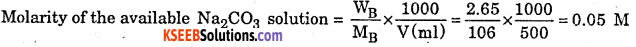For dilution M1V1 = M2V2
⇒ 0.05 × 10 = 1000 × M2
M2 = 0.0005 M (Molarity of the resultant solution).Question 9.
2 L of a solution is prepared by dissolving 0.02 mole of NaBr and 0.02 mole of Na2SO4. 1 L of mixture X + excess of AgNO3 → Y (pale yellow ppt.).
1 L of mixture X + excess of BaCl2 → Z (white ppt.).
What is the ratio of the number of moles of Y and Z?
AgNO3 reacts with Br ions to form AgBr as pale yellow ppt. as per the following reaction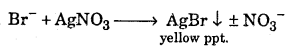2 L of the solution will contain 0.02 mole of NaBr or 0.02 mole of Br.
⇒ 1 L of the solution will contain 0.01 mole of Br
∴ as per equation (i) number of moles of AgBr i.e., Y formed is 0.01 mole
BaCl2 reacts with SO42- ions present in the solution as per the following reaction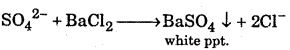2L of solution contain 0.02 mole of Na2SO4 or 0.02 mole of SO42-.
⇒ 1 L of solution will contain 0.01 mole of SO42-.
∴ as per equation (ii) number of moles of BaSO4 i.e., Z formed is 0.01 mole
Ratio of Y and Z = 0.01: 0.01 = 1:1

Question 10.
How many grams of copper will get replaced in 2 L of CuSO4 solution having molarity 1.50 M, if it is made to react with 54 g of aluminium? (At. mass of Cu = 63.5 and A1 = 27.0)
The reaction with CuSO4 solution and aluminium may be represented as
3CUSO4 + 2Al → Al2(SO4)2 +3CU
(3 × 159.5)g = 478.5g (2 × 27)g = 54g
Calculation of wt. of CuSO4 in 2 L of CuSO4 solution having 1.50 molarity478.5 g of CuSO4 reacts with 54 g of aluminium
Also, 159.5 g of CuSO4 has 63.5 g of Cu
1 g of CuSO4 has $$\frac{63.5}{159.5}$$ g of Cu
478.5 g of CuSO4 has $$\frac{63.5}{159.5}$$ × 478.5g of Cu = 190.5g of Cu

Question 11.
0.7 g of Na2CO3.xH2O dissolved to make 100 ml of solution. If 20 ml of this solution is required to completely neutralize 19.8 ml of 0.1 M HCl, then what is the value of x?
Calculation of molarity of sodium carbonate solution
n1M1V1 = n2M2V2 (n factor for sodium carbonate is 2)
2 × 20 × M1 =19.8 × 0.1
⇒ M1 = 0.0495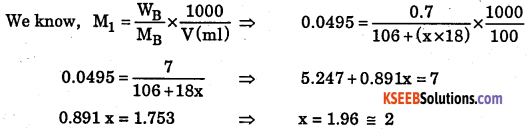The value of x i.e., the number of water molecules of crystallization is 2.

Question 12.
Calculate the weight of CaO that can be obtained by heating 200 kg of limestone which is 95% pure. (At. mass of Ca = 40, C = 12 and O = 16).100 kg of CaCO3 gives 56 kg of CaO.
Since lime stone is 95% pure; amount of CaCO3 present in 200 kg of impure sample = 190 kg CaCO3 100 kg of CaCO3 gives 56 kg of CaO
1 kg of CaCO3 gives $$\frac { 56 }{ 100 }$$kg of CaO
190 kg of CaCO3 give $$\frac { 56 }{ 100 }$$ × 190 = 106.4 kg of CaO.Question 13.
20 g of sample containing Ba(OH)2 is dissolved in 10 ml of 0.5 M HC1 solution. The excess of HCl was then titrated against 0.2 M NaOH. The volume of NaOH used in the titration was 10 ml. Calculate the percentage of Ba(OH)2 in the sample. (Mol. wt. of Ba(OH)2 = 171)
Calculation of volume of HCl used in titration between NaOH and HCl.
VNaOH = 10 ml, MNaOH = 0.2M ,MHCl = 0.5M, VHCl = ?
MNaOH × VNaOH = MHCl × VHCl
VHCl = $$\frac{10 \times 0.2}{0.5}$$ = 4ml
This is the volume of HCl left unused when an excess of HC1 is added to Ba(OH)2 solution.
Total volume of HCl added = 10 ml.
Volume of HCl used to react with Ba(OH)2 = 10 – 4 = 6 ml
∴HCl reacts with Ba(OH)2 as per the equation
2HCl + Ba(OH)2 → BaCl2 + 2H2O
6 ml of 0.5 M HCl has reacted with Ba(OH)2 ⇒ Number of moles of HCl reacted,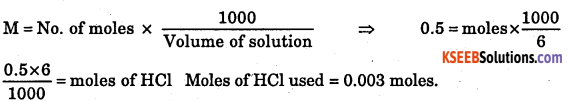Observing the molar ratio of HC1 and Ba(OH)2,
Moles of Ba(OH)2 reacted = $$\frac { 1 }{ 2 }$$ × moles of HCl reacted = $$\frac { 1 }{ 2 }$$ × O.003 = 0.0015 moles
Weight of Ba(OH)2 reacted = no. of moles × mol. wt. = 0.0015× 171 = 0.2565 g### 1st PUC Chemistry Some Basic Concepts of Chemistry Numerical Problems and Answers

Question 1.
How many significant figures are there in each of the following numbers?
(i) 6.005, (ii) 6.002 × 1023, (iii) 8000, (iv) 0.0025, (v) π, (vi) the sum 18.5 + 0.4235, (vii) the product 14 × 6.345.
(i) Four because the zeros between the non-zero digits are significant figures.
(ii) Four because only the first term gives the significant figures and the exponential term is not considered.
(iii) Four. However, if expressed in scientific notation as 8 × 103, it will have only one significant figure, as 8.0 × 103, 8.00 × 103 or 8.000 x 103, it will have 2, 3, or 4 significant figures.
(iv) Two because the zeros on the left of the first non-zero digit are not significant.
(v) As π = $$\frac { 22 }{ 7 }$$ = 3.1428571…., hence it has infinite number of significant figures.
(vi) Three, because the reported sum will be only upto one decimal place i.e., 18.9.
(vii) Two, because the number with the least number of significant figures involved in the calculation (i.e., 14) has two significant figures.

Question 2.
Express the following to four significant figures: (i) 6.45372, (ii) 48.38250, (iii) 70000, (iv) 2.65986 × 103, (v) 0.004687.
(i) 6.454, (ii) 48.38, (iii) 7.000 × 104, (iv) 2.660 × 103, (v) 0.004687.

Question 3.
A sample of nickel weighs 6.5425 g and has a density of 8.8 g cm3 the volume? Report the answer to the correct decimal place.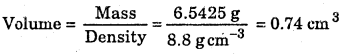The result should have two significant figures because the least precise term (8.8) has two significant figures.Question 4.
Express the result of the following calculation to the appropriate number of $$\frac{3.24 \times 0.08666}{5.006}$$
$$\frac{3.24 \times 0.08666}{5.006}$$= 0.0560883 (Actual result)
As 3.24 has the least number of significant figures, viz., 3, the result should contain 3 significant figures only. Hence, the result will be reported as 0.0561 (after rounding off).

Question 5.
The mass of precious stones is pressed in terms of a carat. Given that 1 carat = 3.168 grains and 1 gram – 15.4 grains, calculate the total mass of the ring in grams and kilograms which contains 0.500 carat diamond and 7.00 gram gold.
The unit conversion factors to be used will be;Question 6.
Two oxides of metal contain 27.6% and 30.0% of oxygen respectively. If the formula of the first oxide is M3O4, find that of the second.
In the first oxide, oxygen = 27.6, metal = 100 – 27.6 = 72.4 parts by mass.
As the formula of the oxide is M3O4, this means 72.4 parts by mass of metal = 3 atoms of metal and 4 atoms of oxygen = 27.6 parts by mass.
In the second oxide, oxygen = 30.0 parts by mass and metal = 100 – 30 = 70 parts by mass.
But 72.4 parts by mass of metal = 3 atoms of metal.
∴ 70 parts by mass of metal =$$\frac{3}{72.4}$$ × 70 atoms of metal = 2.90 atoms of metal.
Also, 27.6 parts by mass of oxygen = 4 atoms of oxygen.
∴ 4.35 atoms of oxygen.
Hence, ratio of M: O in the second oxide = 2.90 : 4.35 = 1:1.5 = 2:3.
∴ Formula of the metal oxide is M2O3.

Question 7.
What is the equivalent weight of KH(IO3)2 as an oxidant in presence of 4.0 (N) HCl when IC becomes the reduced form? (K = 39.0,1 = 127.0).
In KH(IO3)2, IO3 is present as IO3. Oxidation state of I will be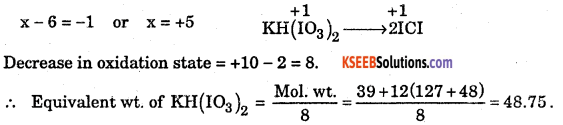Question 8.
Calculate the percentage of the naturally occurring isotopes 35C1 and 37Cl that accounts for the atomic mass of chlorine taken as 35.45.
Suppose 35Cl present = x%. Then 37Cl present = (100 -x)%
∴ Average atomic mass = \frac{x \times 35+(100-x) \times 37}{100} = 35.45 (Given)
or 35x + 3700-37x = 3545 or 2x = 155 or x = 77.5%
This 35Cl = 77.5% and 37Cl = (100 – 77.5) = 22.5%.

Question 9.
Convert 22.4 L into cubic metres.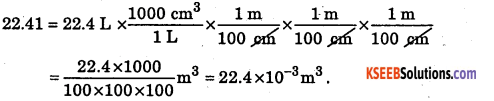Question 10.
Calculate the molar mass of water if it contains 50% heavy water (D2O).
As water contains 50% D2O, this means that it contains $$\frac{1}{2} mole of H20 and \frac{1}{2}$$ mole
of D2O. Mass of $$\frac{1}{2}$$ mole of D2O = $$\frac{1}{2}$$ × (2 × 2 + 16) = 10 g.
Hence, molar mass of the given sample of water = 9 + 10 = 19 g mol-1.

Question 11.
60 cc of oxygen was added to 24 cc of carbon monoxide and the mixture ignited. Calculate the volume of oxygen used up and the volume of carbon dioxide formed.∴ volume of oxygen used up is 12 cc. and the volume of carbon dioxide formed is 24 cc.Question 12.
200 cm3 of carbon monoxide is mixed with 200 cm3 of oxygen at room temperature and ignited. Calculate the vol of CO2 formed on cooling to room temperature. What other gas if any may also be present.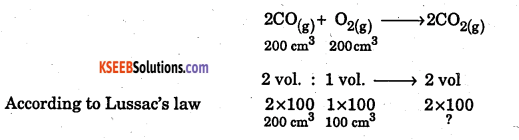Volume of carbon dioxide formed is 200 cm3 and the other gas present is 100 cm3 of oxygen.

Question 13.
Calculate the volume of oxygen required to burn completely a mixture of 22.4 dm3 of CH4 and 11.2 dm3 of H2 (all volumes measured at STP) [1 dm3 = 1 litre].Total oxygen required = 44.8 dm3 +5.6 dm3 =50.4 dm3 Pits].
∴ the number of moles in 7 g of nitrogen is 0.25 moles.

Question 14.
Calculate the mass of 50 cc of CO at STP (C = 12, O = 16).
[1 mole = 1 g mol. wt. and occupies 22.4 lit. at STP]
g mol. wt. of carbon monoxide = 12 + 16 = 28 g
28 g of CO occupies 22400 cc at STP
How many grams of CO will occupy 50 cc at STP = $$\frac{28 \times 50}{22400}$$ = 0.0625 g

Question 15.
Calculate the volume at STP occupied by 6.023 × 1022 molecules of a gas X.
[1 mole of any substance contains 6.023 × 1023 number of molecules [Avogadro’s number]].
(a) 6.022 × 1023 molecules occupied 22.42 cm3 (Its) = $$\frac{22.4 \times 6.023 \times 10^{22}}{6.023 \times 10^{23}}$$ = 2.24 lits

Question 16.
Calculate the number of molecules in 1 kg of sodium chloride. [Na = 23, Cl = 35.5].
(a) 1 mol wt. of NaCl = 58.5 g contains 6.022 × 1023
(b) Contains molecules = $$\frac{1000}{58.5}$$ × 6.023 × 1023 = 102.96 × 1023 molecules.

Question 17.
Calculate the atomicity of a gas X if 1 g of X occupies 11,200 cc at STP [At. Wt. of X= 1].Question 18.
0. 48 g of gas forms 100 cm3 of vapours at STP. Calculate the gram molecular wt. of the gas.
100 cm3 of the gas weighs 0.48 grams at STP
22,400 cm3 weight Mol. wt. at STP.
∴ $$\frac{22400}{100}$$ × 0.48 = 107.52Question 19.
Calculate the weight of a substance X which in gaseous form occupies 10 litres at 27 °C and 700 mm pressure. The molecular Weight of X is 60.
Convert the volume to STP using the gas equation.
Initial conditions: P1 = 700 mm of Hg, V1 = 10 litres, T1 = 27 + 273 = 300 K
Final conditions (at STP): P0 = 760 mm of Hg, V0 = X litres, T0 = 273 K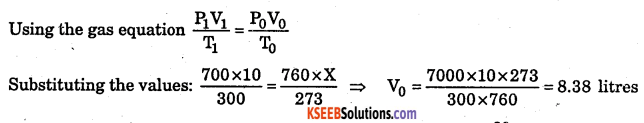Question 20.
Calculate the normality of oxalic acid solution containing 0.895 g crystals in 250 cm3 of its solution.
Molecular formula of oxalic acid crystals = H2C2O4.2H2O = 126Question 21.
25 cm3 of ferrous ammonium sulphate solution require 20 cm3 of 0.1 N potassium dichromate solution. Calculate the amount of ferrous ammonium sulphate crystals dissolved in 250 cm3 of the solution. (Given equivalent)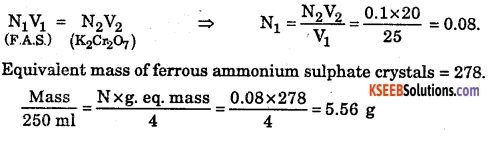Question 22.
What should be the normality of a solution prepared by diluting 250 ml of 0.4 NH2SO4 with 1000 ml of water?
Total volume of the diluted solution = 250 + 1000 = 1250 ml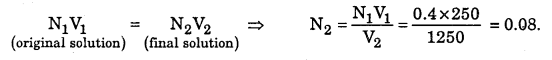Question 23.
20 cm3 of a solution of oxalic acid requires 25 cm3 of 0.2 N potassium permanganate to react completely (a) Calculate the normality of oxalic acid solution, (b) What volume of this oxalic acid solution when made up to 250cm3 gives 0.2 N solution?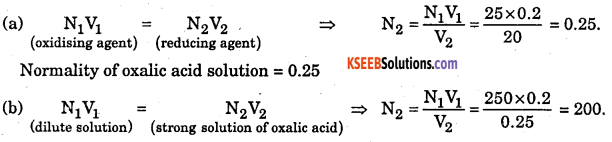Normality of oxalic acid solution = 200.
The volume of oxalic acid solution required = 200 cm3

Question 24.
200 cm3 of a solution of a dibasic acid contains 1.512 g of the acid and the normality of the solution is 0.12. Calculate (i) the equivalent mass and (ii) the molecular mass of the acid.
(i) Mass of the acid in one dm3 of the solution = 1.512 × 5 = 7.56 g.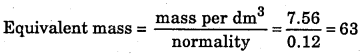(ii) Molecular mass of the acid = eq. mass × basicity = 63 × 2 = 126.

Question 25.
25 cm3 a of a solution of sodium hydroxide required 29 cm3 of $$\frac { n }{ 10 }$$ solution of oxalic acid for neutralization. Find the normality of sodium hydroxide solution and its amount dissolved in 500 cm3 of the solution.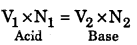Substituting we have 28 × 0.1 = 25 × N2
∴N2 = $$\frac{29 \times 0.1}{25}$$ = 0.116
∴ Normality of sodium hydroxide solution = 0.116
We know that W = N × E for 100 ml
i.e., Mass per dm3 = Normality × eq. nlass = 0.116 × 40 g
∴ Mass of sodium hydroxide in 500 cm 3 of the solution = $$\frac{0.116 \times 40}{2}$$ = 2.32 g.Question 26.
Calculate the volume of concentrated nitric acid of normality 14 required to prepare 1 dm3 of \frac{N}{10} nitric acid.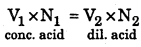Question 27.
Calculate the mass of hydrochloric acid in 200 cm3 of 0.2 N solution of it. What volume of this acid solution will react exactly with 25 cm3 of 0.14 N solution of sodium hydroxide?
Normality of hydrochloric acid = 0.2; Eq. mass of hydrochloric acid = 36.5
Mass of hydrochloric acid in one dm3 of the solution is given by W = N × E = 0.2 × 36.5 g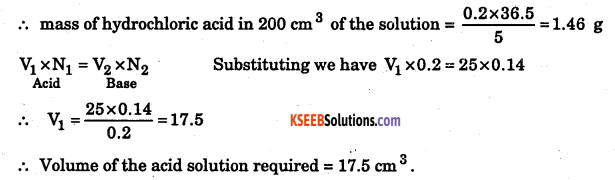Question 28.
0. 99 g of acid was dissolved in water and the solution made up to 200 cm3, 20 cm3 of this solution required 15 cm3 of 0.105 N sodium hydroxide solution for complete neutralization. Find the equivalent mass of the acid.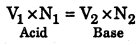Substituting we have 20 × N1 =15 × 0.105
∴ N1 = $$\frac{15 \times 0.105}{20}$$ = 0.07875
∴ Normality of acid solution = 0.07875
Mass of the acid in one dm 3 of the solution – 0.99 × 5 g = 4.95 g
Eq. mass of the acid is given by E = $$\frac{W}{N}=\frac{4.95}{0.07875}$$ = 62.85

Question 29.
2.15 g of impure oxalic acid crystals were dissolved in water and the solution made up to 250 cm3 of this solution required 20 cm3 of 0.16 N sodium hydroxide solution for complete neutalization. What is the percentage purity of oxalic acid crystals?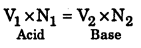Substituting we have 25 × N1 =20 × 0.16
∴ N1 = $$\frac{20 \times 0.16}{25}$$ = 0.128
∴ Normality of oxalic acid solution = 0.128
Eq. mass of oxalic acid crystals H2C2O4.2H2O = 63.
Mass of pure oxalic acid in one dm 3 is given by W = N × E = 0.128 × 63
Mass of pure oxalic in 250 cm3 = $$\frac{0.128 \times 63}{4}$$ = 2.016 g
Mass of impure oxalic acid crystals dissolved in 250 cm3 = 2.15 g
% percentage purity of the given oxalic acid crystals = $$\frac{2.016 \times 100}{2.15}$$ = 93.76Question 30.
A solution of sodium hydroxide contains 4.8 g of the substance per dm3, (a) What volume of the solution is required to prepare 500 cm3 of decinormal solution. (b) What volume of water has to be added to one dm3 of the solution in order to make it exactly decinormal?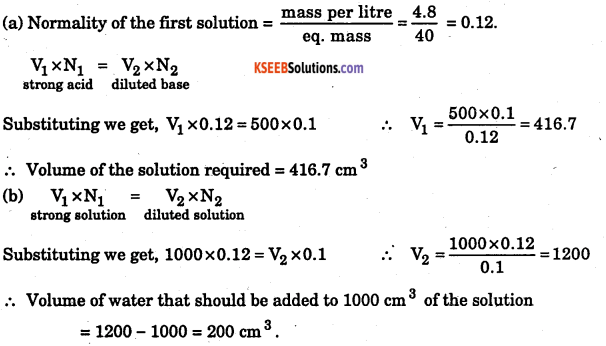Question 31.
Find the Molarity of hydrochloric acid containing 31.5% of hydrochloric acid. Its specific gravity is 1.16.
Mass of 1000 cm3 of the acid = 1000 × 1.16 = 1160 g
Mass of hydrochloric acid in 100 g of the solution = 3.15 g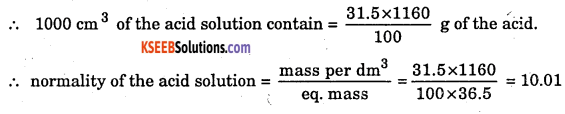Question 32.
100 cm3 of concentrated hydrochloric acid which is 11 M is diluted to 275 cm3. If 2.5 g of limestone are required to neutralize 10 cm3 of this diluted – acid, what is the percentage of calcium carbonate in the sample of limestone?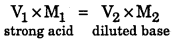Substituting we get, 100 × 11 = 275 × M2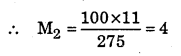∴ Normality of the diluted acid = 4
1000 cm3 of the acid solution contain 4 gram
∴ 10 cm3 of the diluted acid contain = $$\frac{4 \times 10}{1000}$$ i.e., 0.4 gram
2.5 g of limestone contain 0.04 gram of calcium carbonate i.e., they contain 0.04 × 100 g = 4 g of calcium carbonate.
(Molar mass of calcium carbonate =100)
∴ percentage of calcium carbonate in the sample of limestone = $$\frac{4 \times 100}{2.5}$$ = 16.0

Question 33.
25.0 cm3 of acid required exactly 20.5 cm3 of deci molar base for complete neutralization. What is the normality of the acid?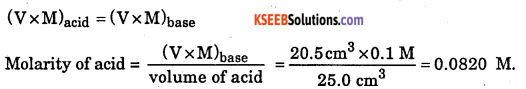Question 34.
Exactly 20.0 cm3 of nitric acid neutralized 28.4 cm3 of 0.25 M NaOH. What is the molarity of the acid?